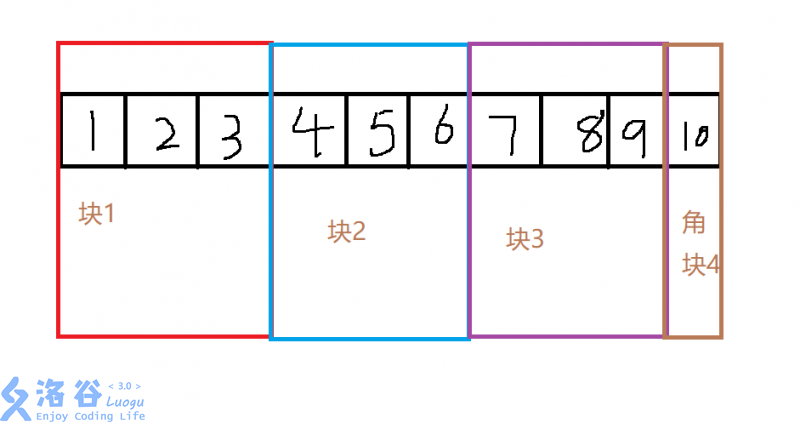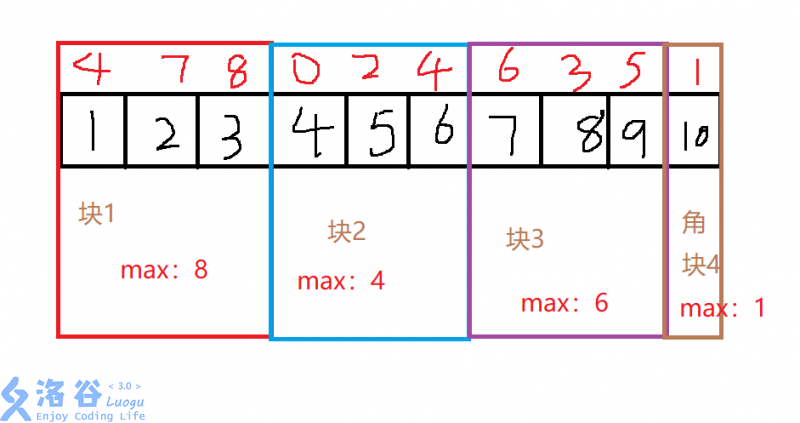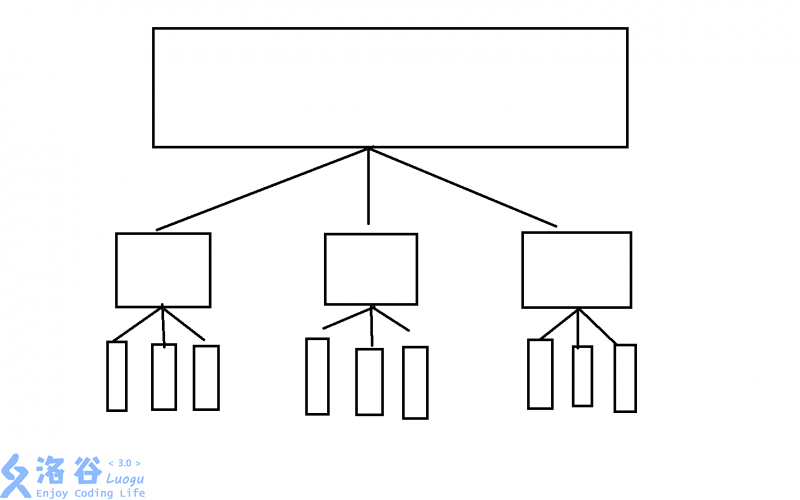### 基础根号算法—分块

check评论：0 条 remove_red_eye浏览量：588 change_historyTags：编程学习笔记int n;//总个数
int block=sqrt(n);//每一块大小
for(int i=1;i<=n;i++)
{
belong[i]=(i-1)/block+1;//每一个数所在块
}

给定一个长的为n数列，求出任意区间[l,r]的最大值(1<=l,r<=n)(l<=r)## 分块的实质## 分块算法的延申

• 莫队• 带修莫队

/*基础莫队操作*/
while(l<Q[i].x) Delet(a[l++]);
while(r>Q[i].y) Delet(a[r--]);
/*基础莫队操作*/
/*继续修改的操作*/
while(now<Q[i].pre) Work(++now,i);
while(now>Q[i].pre) Work(now--,i);
/*修改回去的操作*/
out[Q[i].id]=ans;//统计答案


#### PS：洛谷P3380 二逼平衡树第一篇题解居然是分块写的欸qwq

chevron_left 浅析FFT与NTT#### 暂无评论